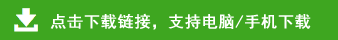# 小学四年级数学下册《课前预习单》

小学四年级数学下册《课前预习单》部分内容预览

1　加减法的关系

 项目 内容 1.根据每组中的三个数各写出四个算式。(1)3、7、10(2)4、5、9 2.读教材第2~3页例题。分析与解答:(1)这是已知两段铁路分别长多少千米,求把它们合起来是多少千米。应该用加法计算,列式计算为814+1142=()。(2)已知西宁到拉萨的铁路全长1956 km,其中西宁到格尔木长814 km,求格尔木到拉萨的铁路长多少千米,用减法计算,列式计算为1956-814=()。(3)已知西宁到拉萨的铁路全长1956 km,其中格尔木到拉萨长1142 km,求西宁到格尔木的铁路长多少千米,也用减法计算,列式计算为1956-1142=()。 3.通过预习,我知道了和=加数+加数,加数=()-另一个加数;差=()-减数,减数=被减数-(),被减数=()+差。4.通过预习,我还知道:减法是加法的逆运算,验算加法可以用()。 5.列竖式计算,并验算。136+293=328+491=621+183= 416-172=438-274=439+280= 417-183=551+265= 温馨提示 知识准备:加减法之间的互逆关系、加减运算中的三个量之间的关系。

1　加减法的关系
1.(1)3+7=10　7+3=10　10-3=7　10-7=3
(2)4+5=9　5+4=9　9-4=5　9-5=4
2.(1)1956　(2)1142
(3)814
3.和　被减数　差　减数
4.减法　429　819　804　244　164　719　234　816